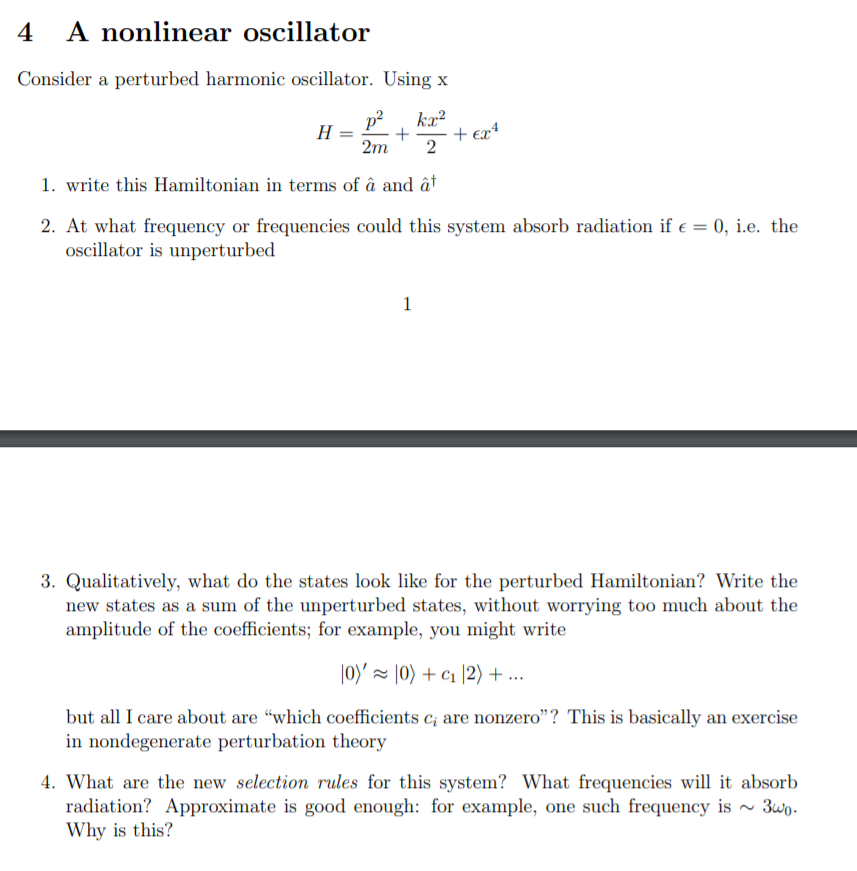# 4 A nonlinear oscillator Consider a perturbed harmonic oscillator. Using x p2 H + ke? 2...

###### Question:4 A nonlinear oscillator Consider a perturbed harmonic oscillator. Using x p2 H + ke? 2 + ex4 2m 1. write this Hamiltonian in terms of â and at 2. At what frequency or frequencies could this system absorb radiation if € = 0, i.e. the oscillator is unperturbed 1 3. Qualitatively, what do the states look like for the perturbed Hamiltonian? Write the new states as a sum of the unperturbed states, without worrying too much about the amplitude of the coefficients; for example, you might write 10)' — \0) + C1 2) + ... but all I care about are “which coefficients c; are nonzero”? This is basically an exercise in nondegenerate perturbation theory 4. What are the new selection rules for this system? What frequencies will it absorb radiation? Approximate is good enough: for example, one such frequency is ~ 3wo. Why is this?